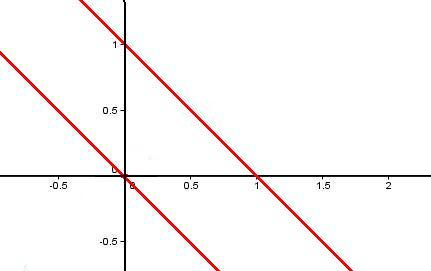You may also likePower Up

Show without recourse to any calculating aid that 7^{1/2} + 7^{1/3} + 7^{1/4} < 7 and 4^{1/2} + 4^{1/3} + 4^{1/4} > 4 . Sketch the graph of f(x) = x^{1/2} + x^{1/3} + x^{1/4} -xParabella

This is a beautiful result involving a parabola and parallels.Snookered

In a snooker game the brown ball was on the lip of the pocket but it could not be hit directly as the black ball was in the way. How could it be potted by playing the white ball off a cushion?

Spot the Difference

Age 16 to 18 ShortChallenge Level

I used a graphical package to draw these 3 pairs of curves on the same set of axes:

$x + y -1 =0\quad\mbox{and}\quad (x+y-1)(x^2+y^2)=0$

$x + y = 0\quad\mbox{and}\quad (x + y)(y^2+(x+1)^2)=0$

$x + y = 1\quad\mbox{and}\quad x^3 + 3xy + y^3 = 1$

I was surprised to find that only 2 graphs appeared to show on the output:Can you explain what has happened? Can you work out which curves go with which lines? Are there any points missing from the graphs? If so, where should these be?

Can you think of any others sets of curves which might fool the computer?